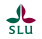Show all terms

## Logarithms and dilutions

### Introduction

Logarithms (log) are often used to simplify calculations with large numbers. When discussing the number of microorganisms in cultures from for instance food samples or when preparing and describing dilutions, logarithms are most often used to express the dilution factor.

### Calculations

The logarithm (log) of a certain number (x) is the number (y) to which another number (b) must be raised, to give the number x. That is:

x = by

The number b is called the base in the logarithmic system you want to use and most common in science and engineering is that you use the number 10 as a base and then you talk about base-10, decimal or common logarithms, which are usually written log10 or lg10. For the sake of simplicity, only log or lg is often written or said, when referring to the base-10 logarithm. An example:

lg 1000 = 3 because 1000 = 10x10x10, which can also be written 103.

According to the logarithm rules, e.g. the following expressions apply for all types of logarithms:

1.     log (a x b) = log a + log b
2.     log (a / b) = log a - log b
3.     log ap = p log a

It follows from the second rule  that log10 (1 / b) = 0-log10b = -log10b

I.e. logarithms can also be negative, e.g.:

log10 (1/1000) = -3, since log10 (1/1000) = 0-3 = -3

In other words:
...
103 = 1000
102 = 100
101 = 10
100 = 1 (can not really be explained mathematically, but has for practical reasons been defined as 1) )
10-1 = 0.1
10-2 = 0,01
10-3 = 0.001
...

### Dilutions

In microbiology, one often wants to make dilution series when deciding how many microorganisms there are in a sample. The most common is to make a 10-step dilution series to achieve high accuracy at large dilutions. This means that the dilution factor is 1:10 in each step and that from one sample you take 1 part of sample solution to 9 parts of culture medium (or another suitable liquid) in a test tube, mix and transfer 1 part of mixture to 9 parts of culture medium in a new test tube  and so on until a suitable degree of dilution has been reached. Then you will get a series of test tubes with dilution factors 1:10, 1: 100, 1: 1000, 1:10 000 and so on. These dilution factors can also be written as: 1/10, 1/100, 1/1000, 1/10 000 and so on, or 0.1, 0.01, 0.001, 0.0001. This can also be expressed with the help of logarithms, i.e. 10-1, 10-2, 10-3, 10-4 and so on.

Therefore, a 1:1000 dilution is sometimes casually referred to as a minus-three dilution.

Read more about how to use dilution series to determine bacterial amounts under Colony Forming Unit (CFU).

Other logarithms

Logarithms with bases other than 10 are used for practical reasons in different disciplines.

Binary logarithms (lb) with base 2 are used in e.g. computer science, bioinformatics and photography.

Natural logarithms (ln) with base e [a transcendental number (limit value), which is approximately equal to 2.71828 ...] are used in e.g. mathematics, physics and chemistry.

Updated: 2021-06-23.

## Recent blog posts